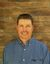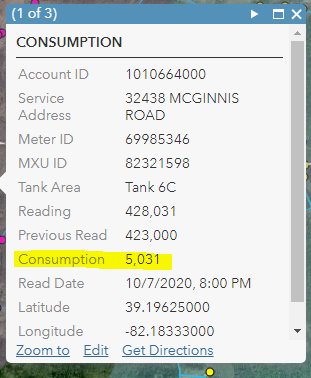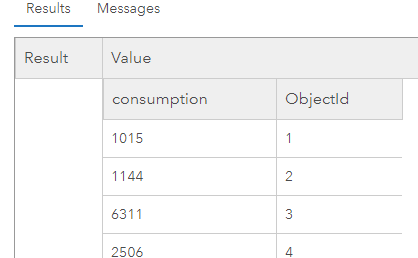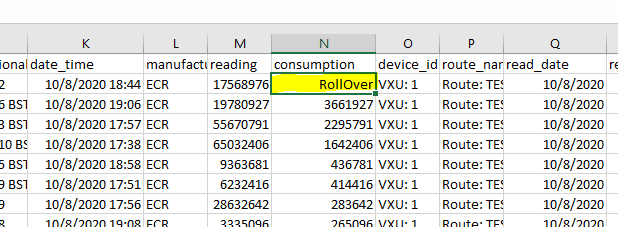# Arcade: totaling field values of features contained within a polygon.

3854
12
10-22-2020 08:54 AMOccasional Contributor

This is related to water consumption and waterloss to add context. I have the following two layers in my map:

-Zones

-Meters

I've been trying to make this work in Arcade, but can't exactly figure out how. I have figured out how to Count the meters contained in the "zone" as shown below in the map with the pop-up, but I also want to display the total of the field "Consumption" of all the meters contained in that polygon/zone. So if I had a Zone and there were 5 meters in the zone, and each customer consumed 1,000 gallons, I want to display 5,000 gallons on the pop-up for that Zone.

var meter = FeatureSetByName(\$map,"METERS")
var CountMeters = Count(Intersects(meter, \$feature))
Return CountMeters

I'm focused on using the "(Intersects(meter, \$feature))" with the function of SUM, but I'm not sure that's the right direction. In words, I want to find all the features from the Meter layer that are contained in the polygon Zone, and then total the attribute field "consumption" of those objects. I'm looking for the total water consumption within that Zone. I can only find examples of counting objects using the Intersect function, but not totaling fields using the Intersect function.

Any help would be greatly appreciated.

Attibute table for a Meter:DMA Zone and Meters:1 Solution

Accepted SolutionsbyEsri Esteemed Contributor

You should be able to use the Sum function to do just that:

``````// access the meters featureset, limiting the fields to Consumption
var meters = FeatureSetByName(\$map, "METERS", ["Consumption"], False);

// count the meters
var CountMeters = Count(Intersects(meters, \$feature));

// Calculate consumption
var consumption = 0;

// check to see if there are meters in the zone
if (CountMeters > 0) {
// calculate the sum of the consumption
consumption = Sum(meters , "Consumption");
}

// return the result
Return consumption;‍‍‍‍‍‍‍‍‍‍‍‍‍‍‍‍‍``````

You can also include the number of meters found in the resulting text if that is something you want:

``````// access the meters featureset, limiting the fields to Consumption
var meters = FeatureSetByName(\$map, "METERS", ["Consumption"], False);

// count the meters
var CountMeters = Count(Intersects(meters, \$feature));

// Calculate consumption
var consumption = 0;
var result = "";

// check to see if there are meters in the zone
if (CountMeters > 0) {
// calculate the sum of the consumption
consumption = Sum(meters , "Consumption");
result = consumption + " gallon (" + CountMeters + " meters in zone)";
} else {
result = "0 gallons (no meters in zone)";
}

// return the result
return result;
‍‍‍‍‍‍‍‍‍‍‍‍‍‍‍‍‍‍‍‍‍``````
12 RepliesbyEsri Esteemed Contributor

You should be able to use the Sum function to do just that:

``````// access the meters featureset, limiting the fields to Consumption
var meters = FeatureSetByName(\$map, "METERS", ["Consumption"], False);

// count the meters
var CountMeters = Count(Intersects(meters, \$feature));

// Calculate consumption
var consumption = 0;

// check to see if there are meters in the zone
if (CountMeters > 0) {
// calculate the sum of the consumption
consumption = Sum(meters , "Consumption");
}

// return the result
Return consumption;‍‍‍‍‍‍‍‍‍‍‍‍‍‍‍‍‍``````

You can also include the number of meters found in the resulting text if that is something you want:

``````// access the meters featureset, limiting the fields to Consumption
var meters = FeatureSetByName(\$map, "METERS", ["Consumption"], False);

// count the meters
var CountMeters = Count(Intersects(meters, \$feature));

// Calculate consumption
var consumption = 0;
var result = "";

// check to see if there are meters in the zone
if (CountMeters > 0) {
// calculate the sum of the consumption
consumption = Sum(meters , "Consumption");
result = consumption + " gallon (" + CountMeters + " meters in zone)";
} else {
result = "0 gallons (no meters in zone)";
}

// return the result
return result;
‍‍‍‍‍‍‍‍‍‍‍‍‍‍‍‍‍‍‍‍‍``````Occasional Contributor

Xander,

I was hoping you would reply. You really seem to know this well.

I'll go through you're example and see if I can mirror that into my map. The ["Consumption"] was the piece I was missing in your following line of code.

``var meters = FeatureSetByName(\$map, "METERS", ["Consumption"], False);``

One quick question; what does the False do at the end of that above line?

Thanks again Xander.

TravisbyEsri Esteemed Contributor

The "False" parameter defines if you want to include the geometry in the result yes or no. In this case it doesn't. Here is a direct link to the documentation: https://developers.arcgis.com/arcade/function-reference/data_functions/#featuresetbyname

[optional] indicates whether to include the geometry in the features. By default, this is true. For performance reasons, you should only request the geometry if necessary, such as for use in geometry functions.

This can be little confusing, since after retrieving the featureset you use Intersects to get the meters in the DMA zone. Even with the include geometry option set to false you will be able to do the Intersects correctly. Only when you want to access the individual geometries and do spatial operations on them you will need to include them in the FeatureSetBy* function.Occasional Contributor

Xander,

I tried to insert the lines of code into Arcade, but I receive an Execution Error:Error. I pasted the code below. The only difference is I deleted my original layer called Meters so I could start clean and replaced it with Services. The entire table/fields are exactly the same with the field Consumption containing the values that I want to total.

I test each line as I go and to see it they return values. For example, I commented out all the lines and just ran the line starting with var meters = FeatureSetByName(\$map, "Services", ["Consumption"], False) and added return meters. Pasted below is a snippet of the results, so I know it's working.When I uncomment the if statement is when I get the error. I guess I'm not following how the code knows which meters are contained in the feature to use in the Sum calculation. I understand that Count(...) counts the objects contained in the intersection, but I'm not sure how those particular meters are then passed to the function Sum to only calculate the consumption of meters contained within Interesection. It seems that Count only returns the number of Objects contained in the intersection. If I Return CountMeters I just get a single integer. If I go through the if statement, and CountMeters = 10, which is greater than 0, which seems to be a check to insure there are meters, but seems like it would Sum the consumption field for all meters, not the ones contained in the Intersection. Would that be correct?

1. // access the meters featureset, limiting the fields to Consumption
2. var meters = FeatureSetByName(\$map, "Services", ["Consumption"], False);
3. // count the meters
var CountMeters = Count(Intersects(meters, \$feature));
4. // Calculate consumption
var consumption = 0;
5. // check to see if there are meters in the zone
if (CountMeters > 0) {
6. // calculate the sum of the consumption
consumption = Sum(meters , "Consumption");
}
7. // return the result
Return consumption;

Using Test, I tried to compile these lines code to see what would happen:

var meters = FeatureSetByName(\$map, "Services", ["Consumption"], False);

consumption = Sum(meters , "Consumption");

Return consumption

The following also gives me that same error Execution Error:Error.

consumption = Sum(meters , "Consumption");

So I think it has more to do with the Sum function then the if statement.

Any ideas?byEsri Esteemed Contributor

You are absolutely right about your observation that the sum is not taking the intersected features but all the features (meters). My bad. Sorry. The code should have been like this (creating a featureset "fs" with the intersected features and use that featureset on line 17 to sum the consumption):

``````// access the meters featureset, limiting the fields to Consumption
var meters = FeatureSetByName(\$map, "Services", ["Consumption"], False);

// intersect the meters or services with the DMA
var fs = Intersects(meters, \$feature);

// count the meters
var CountMeters = Count(fs);

// Calculate consumption
var consumption = 0;
var result = "";

// check to see if there are meters in the zone
if (CountMeters > 0) {
// calculate the sum of the consumption
consumption = Sum(fs , "Consumption");
result = consumption + " gallon (" + CountMeters + " meters in zone)";
} else {
result = "0 gallons (no meters in zone)";
}

// return the result
return result;
‍‍‍‍‍‍‍‍‍‍‍‍‍‍‍‍‍‍‍‍‍‍‍‍``````

What I don't understand is why in the code you shared, it results in an execution error. Is there any additional information available?

The reason why the other snippet is resulting in an error is the missing "var" at the beginning of line 2:

``````var meters = FeatureSetByName(\$map, "Services", ["Consumption"], False);
var consumption = Sum(meters , "Consumption");
Return consumption;‍‍‍``````

Please always include the actual error message, since this contains important information in order to understand the error.

It may also be good to validate if there are any invalid values in the Consumption field that are causing the expression to fail.Occasional Contributor

Xander,

I still receive the same Execution Error:Error. I pasted below what I'm executing, but it should be exactly what you outlined. I'm just pasting it in. If I go through the same process as before and comment lines out and Test the code as I go, it works until I get the Sum function.

1. // access the meters featureset, limiting the fields to Consumption
2. var meters = FeatureSetByName(\$map, "Services", ["Consumption"], False);
3. // intersect the meters or services with the DMA
var fs = Intersects(meters, \$feature)
4. // count the meters
var CountMeters = Count(fs);
5. // Calculate consumption
var consumption = 0;
var result = " ";
6. // check to see if there are meters in the zone
if (CountMeters > 0) {
7. // calculate the sum of the consumption
consumption = Sum(fs, "Consumption");
result = consumption + " gallon (" + CountMeters + " meters in zone)";
} else {
8. result = "0 gallons (no meters in zone)";
}
9. // return the result
return result;

If I simply wanted to Sum the Consumption Field for the layer Services, would it be this:

1. var meters = FeatureSetByName(\$map, "Services", ["Consumption"], False);  (no error)
2. var usage = 0;    (no error)
3. var usage = Sum(meters, "Consumption");   ("Execution Error: Error" when uncommented)
4. Return usage;

It seems like there's something about that Sum function that it does not like. The actual field name is "consumption", lower case, but are things case sensitive? Or do double quotes mean something different than single quotes, or bracket, etc? Sorry for another reply. I know this is really close to working.

TravisbyEsri Esteemed Contributor

Is there some way that you share a portion of the data with me? Perhaps send it to "xbakker [at] esri [dot] co" I just did something similar on some local data and it all seems to work. This might be data related. I can assume that the Consumption field is numeric, right? As far as I can see it does not matter if you use uppercase or lowercase for the field name.byEsri Esteemed Contributor

It seems that for some reason the consumption field in the services featureclass was renamed to "consumptio" (without the "n"). If you change that twice in the code (lines 2 and 17) it should work:

``````// access the meters featureset, limiting the fields to Consumption
var meters = FeatureSetByName(\$map, "Services", ["Consumptio"], False);

// intersect the meters or services with the DMA
var fs = Intersects(meters, \$feature);

// count the meters
var CountMeters = Count(fs);

// Calculate consumption
var consumption = 0;
var result = "";

// check to see if there are meters in the zone
if (CountMeters > 0) {
// calculate the sum of the consumption
consumption = Sum(fs , "Consumptio");
result = consumption + " gallon (" + CountMeters + " meters in zone)";
} else {
result = "0 gallons (no meters in zone)";
}

// return the result
return result;‍‍‍‍‍‍‍‍‍‍‍‍‍‍‍‍‍‍‍‍‍‍‍‍``````

Result:Occasional Contributor

Thanks for the help Xander, I really appreciate it.

I was working on this over the weekend, and was still getting an error after trying your suggestion in the above reply. It made no sense. I decided I would try another field in the attribute table to see what would occur I used the "reading" field which was the current reading of the meter. Worked perfectly. Changed it back to the "consumption" field and it would give me an error. Deleted everything, started a new map, reloaded the layers, same result. Just by chance I sorted the table I was uploading to AGOL and there it was:In our meter reading software when a meter rolls over (9,999,999 goes back to zero), it throws out this alert to let us know that it occurred and we need to adjust the reading.  When I changed "RollOver" to a number it worked exactly as it should. Pretty frustrating.

Can I ask one more question? I'm not sure of the best way to do this, but pertains to the waterloss calculation I need to make. It is simply what the customers consumed (consumption) subtracted from the master/zone meter. The customer meters are in each zone and I could include the master/zone meter within that zone, or leave it out. All the customer meters have the same zone name, but the master/zone meter is named different:

customer meter zone name= Tank 6C

master meter zone name = 6C Bolin Road

If I leave the master/zone meter within the zone, it will be included in the sum of "consumption" and the total would not be correct. If I leave it out, the sum of "consumption" would be correct, but I would still need to subtract that total from the master/zone meter "consumption" to get waterloss. I'm not sure how to pull or use that "consumption" value of only the master meter. I thought FILTER may work by using the name, but I still don't know how I would pull the consumption value. I thought I might be able to use the MAX function to pull the value of the master/zone meter, but I think then I would have to include the master meter within the zone. I can't quite wrap my head around what to do to make it work.

I then have the particular case where I have "Master Meter A" that feeds "Customer meters A", but also feeds Master Meter B, and customer meters B. In this case to get water loss I would be: Waterloss in Zone A  = Master Meter A - Customer A - Master Meter B. Now I'm dealing with two different zones adding to the complexty. I'm sure there's a way to go about it though.

Thanks Xander.

Travis